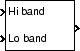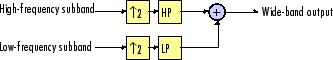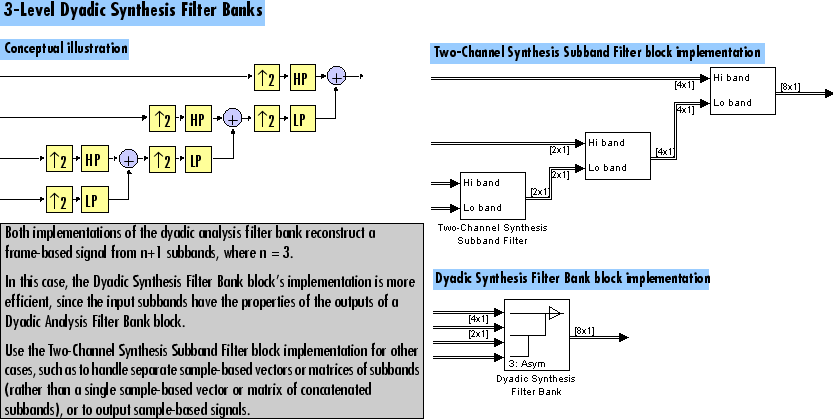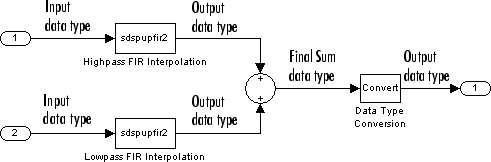# Two-Channel Synthesis Subband Filter

Reconstruct signal from high-frequency and low-frequency subbands

## Library

Filtering / Multirate Filters

`dspmlti4`

•## Description

The Two-Channel Synthesis Subband Filter block reconstructs a signal from its high-frequency and low-frequency subbands, each with half the bandwidth and half the sample rate of the original signal. Use this block to reconstruct signals decomposed by the Two-Channel Analysis Subband Filter block.

The block upsamples the high- and low-frequency subbands by 2, and then filters the results with a pair of highpass and lowpass FIR filters, as illustrated in the following figure.The block implements the FIR filtering and downsampling steps together using a polyphase filter structure, which is more efficient than the straightforward interpolate-then-filter algorithm shown in the preceding figure. You can implement a multilevel dyadic synthesis filter bank by connecting multiple copies of this block or by using the Dyadic Synthesis Filter Bank block. For more information, see Creating Multilevel Dyadic Synthesis Filter Banks.

You must provide a vector of filter coefficients for the lowpass and highpass FIR filters. Each filter should be a half-band filter that passes the frequency band that the other filter stops. You can use this block to reconstruct the output of a Two-Channel Analysis Subband Filter block. To do so, you must design the filters in this block such that they perfectly reconstruct the outputs of the analysis filters.

### Specifying the FIR Filters

You must provide the vector of numerator coefficients for the lowpass and highpass filters in the Lowpass FIR filter coefficients and Highpass FIR filter coefficients parameters.

For example, to specify a filter with the following transfer function, enter the vector `[b(1) b(2) ... b(m)]`.

`$H\left(z\right)=B\left(z\right)={b}_{1}+{b}_{2}{z}^{-1}+\dots +{b}_{m}{z}^{-\left(m-1\right)}$`

Each filter should be a half-band filter that passes the frequency band that the other filter stops. You can use this block to reconstruct the output of a Two-Channel Analysis Subband Filter block. To do so, you must design the filters in this block such that they perfectly reconstruct the outputs of the analysis filters.

The best way to design perfect reconstruction filters is to use the Wavelet Toolbox™ `wfilters` function for the filters in both this block and in the corresponding Two-Channel Analysis Subband Filter block. You can also use DSP System Toolbox™ and Signal Processing Toolbox™ functions.

The Two-Channel Synthesis Subband Filter block initializes all filter states to zero.

### Frame-Based Processing

When you set the Input processing parameter to ```Columns as channels (frame based)```, the block accepts any two M-by-N matrices with the same frame rates. The block treats each column of the input as the high- or low-frequency subbands of the corresponding output channel. You can use the Rate options parameter to specify how the block resamples the input:

• When you set the Rate options parameter to ```Enforce single-rate processing```, the input to the block can be any two M-by-N matrices with the same frame rate. The block treats each input column as the high- or low-frequency subbands of the corresponding output channel. The input to the topmost input port should contain the high-frequency subbands. The block outputs one matrix, where each column is reconstructed from the corresponding columns of each input matrix. The input and output frame rates are the same, but the frame size of the output is twice that of the input.

• When you set the Rate options parameter to ```Allow multirate processing```, the block treats each column of the input as the high- or low-frequency subbands of the corresponding output channel. The input to the topmost input port should contain the high-frequency subbands. The block outputs one matrix, where each column is reconstructed from the corresponding columns of the input matrices. The input and output frame sizes are the same, but the frame rate of the output is twice that of the input. Thus, the overall sample rate of the output is twice that of the input sample rate.

In this mode, the block has one frame of latency, as described in the Latency section.

### Sample-Based Processing

When you set the Input processing parameter to `Elements as channels (sample based)`, the block accepts any two M-by-N matrices with the same sample rates. The block treats each M-by-N matrix as M · N independent subbands. Each element of the input matrices is the high- or low-frequency subband of the corresponding channel in the output matrix. The input to the topmost input port should contain the high-frequency subbands. The block outputs one matrix with the same dimensions as the input matrices, but a sample rate that is twice that of the input. The block reconstructs each element of the output from the corresponding elements in the input matrices.

Depending on the setting of your Simulink® configuration parameters, the output may have one sample of latency, as described in the Latency section.

### Latency

When you set the Input processing parameter to ```Columns as channels (frame based)``` and the Rate options parameter to `Enforce single-rate processing`, the Two-Channel Synthesis Subband Filter block always has zero-tasking latency. Zero-tasking latency means that the block propagates the first input sample (received at time t= 0) as the first output sample.

When you set the Rate options parameter to ```Allow multirate processing```, the Two-Channel Synthesis Subband Filter block may exhibit latency. The amount of latency depends on the setting of the Input processing parameter of this block and the setting of the Simulink Treat each discrete rate as a separate task configuration parameter. The following table summarizes the conditions that produce latency when the block is performing multirate processing.

Input processingTreat each discrete rate as a separate taskLatency

```Elements as channels (sample based)```

`Off`

None.

`On`

One sample. The first output sample in each channel always has a value of `0`.

```Columns as channels (frame based)```

`Off` or `On`

One frame. All samples in the first output frame have a value of `0`.

Note

### Creating Multilevel Dyadic Synthesis Filter Banks

The Two-Channel Synthesis Subband Filter block is the basic unit of a dyadic synthesis filter bank. You can connect several of these blocks to implement an n-level filter bank, as illustrated in the following figure. For a review of dyadic synthesis filter banks, see the Dyadic Synthesis Filter Bank block reference page.

When you create a filter bank by connecting multiple copies of this block, the output values of the filter bank differ depending on whether there is latency. Though the output values differ, both sets of values are valid; the difference arises from changes in latency. See the Latency section for more information about when latency can occur in the Two-Channel Analysis Subband Filter block.

In some cases, rather than connecting several Two-Channel Analysis Subband Filter blocks, you can use the Dyadic Analysis Filter Bank block, which is faster and requires less memory. In particular, the Dyadic Analysis Filter Bank block is more efficient under the following conditions:

• You are reconstructing a signal from 2n or n+ 1 subbands.

• The frame size of the signal you are reconstructing is a multiple of 2n.

• The properties of the subbands you are working with match those of the outputs of the Dyadic Analysis Filter Bank block. These properties are described in the Dyadic Analysis Filter Bank reference page.The Dyadic Synthesis Filter Bank block allows you to specify the filter bank filters by providing vectors of filter coefficients, just as this block does. The Dyadic Synthesis Filter Bank block provides an additional option of using wavelet-based filters that the block designs by using a wavelet you specify.

### Fixed-Point Data Types

The Two-Channel Synthesis Subband Filter block is composed of two FIR Interpolation blocks as shown in the following diagram.For fixed-point signals, you can set the coefficient, product output, accumulator, and output data types used in the FIR Interpolation blocks as discussed in Parameters. For a diagram showing the usage of these data types within the FIR blocks, see the FIR Interpolation block reference page.

In addition, the inputs to the Sum (Simulink) block shown in the previous diagram are accumulated using the accumulator data type. The output of the Sum block is then cast from the accumulator data type to the output data type. Therefore the output of the Two-Channel Synthesis Subband Filter block is in the output data type. You also set these data types in the block dialog box as discussed in the Parameters section.

## Parameters

Main Tab

Lowpass FIR filter coefficients

A vector of lowpass FIR filter coefficients, in descending powers of z. The lowpass filter should be a half-band filter that passes the frequency band stopped by the filter specified in the Highpass FIR filter coefficients parameter. To use this block to reconstruct the output of a Two-Channel Analysis Subband Filter block, you must design the filters in this block to perfectly reconstruct the outputs of the analysis filters. For more information, see Specifying the FIR Filters.

Highpass FIR filter coefficients

A vector of highpass FIR filter coefficients, in descending powers of z. The highpass filter should be a half-band filter that passes the frequency band stopped by the filter specified in the Lowpass FIR filter coefficients parameter. To use this block to reconstruct the output of a Two-Channel Analysis Subband Filter block, you must design the filters in this block to perfectly reconstruct the outputs of the analysis filters. For more information, see Specifying the FIR Filters.

Input processing

Specify how the block should process the input. You can set this parameter to one of the following options:

• `Columns as channels (frame based)` (default) — When you select this option, the block treats each column of the input as a separate channel.

• `Elements as channels (sample based)` — When you select this option, the block treats each element of the input as a separate channel.

Rate options

Specify the rate processing rule for the block. You can set this parameter to one of the following options:

• `Enforce single-rate processing` — When you select this option, the block treats each column of the input as an independent channel and reconstructs each channel over time. The output has the same sample rate as the input, but the output frame size is twice that of the input frame size. To select this option, you must set the Input processing parameter to `Columns as channels (frame based)`.

• `Allow multirate processing` — When you select this option, the input and output of the block are the same size, but the sample rate of the output is twice that of the input.

Some settings of this parameter cause the block to have nonzero latency. See Latency for more information.

Data Types Tab

Rounding mode

Select the rounding mode for fixed-point operations. The filter coefficients do not obey this parameter; they always round to `Nearest`.

Note

The Rounding mode and Saturate on integer overflow settings have no effect on numerical results when all the following conditions exist:

• Product output is ```Inherit: Inherit via internal rule```

• Accumulator is ```Inherit: Inherit via internal rule```

• Output is ```Inherit: Same as accumulator```

With these data type settings, the block is effectively operating in full precision mode.

Saturate on integer overflow

When you select this parameter, the block saturates the result of its fixed-point operation. When you clear this parameter, the block wraps the result of its fixed-point operation. For details on `saturate` and `wrap`, see overflow mode for fixed-point operations.

Note

The Rounding mode and Saturate on integer overflow parameters have no effect on numeric results when all these conditions are met:

• Product output data type is ```Inherit: Inherit via internal rule```.

• Accumulator data type is ```Inherit: Inherit via internal rule```.

With these data type settings, the block operates in full-precision mode.

Coefficients

Specify the coefficients data type. See Fixed-Point Data Types and Multiplication Data Types for illustrations depicting the use of the coefficients data type in this block. You can set it to:

• A rule that inherits a data type, for example, ```Inherit: Same word length as input```

• An expression that evaluates to a valid data type, for example, `fixdt(1,16,0)`

Click the buttonto display the Data Type Assistant, which helps you set the Coefficients parameter.

Coefficients Minimum

Specify the minimum value of the filter coefficients. The default value is `[]` (unspecified). Simulink software uses this value to perform:

• Automatic scaling of fixed-point data types

Coefficients Maximum

Specify the maximum value of the filter coefficients. The default value is `[]` (unspecified). Simulink software uses this value to perform:

• Automatic scaling of fixed-point data types

Product output

Specify the product output data type. See Fixed-Point Data Types and Multiplication Data Types for illustrations depicting the use of the product output data type in this block. You can set it to:

• A rule that inherits a data type, for example, ```Inherit: Inherit via internal rule```. For more information on this rule, see Inherit via Internal Rule.

Note

The actual product output word length may be equal to or greater than the calculated ideal product output word length, depending on the settings on the Hardware Implementation pane of the Configuration Parameters dialog box.

• An expression that evaluates to a valid data type, for example, `fixdt(1,16,0)`

Click the buttonto display the Data Type Assistant, which helps you set the Product output parameter.

Accumulator

Specify the accumulator data type. See Fixed-Point Data Types for illustrations depicting the use of the accumulator data type in this block. You can set this parameter to:

• A rule that inherits a data type, for example, ```Inherit: Inherit via internal rule```. For more information on this rule, see Inherit via Internal Rule.

• An expression that evaluates to a valid data type, for example, `fixdt(1,16,0)`

Click the buttonto display the Data Type Assistant, which helps you set the Accumulator parameter.

Output

Specify the output data type. See Fixed-Point Data Types for illustrations depicting the use of the output data type in this block. You can set it to:

• A rule that inherits a data type, for example, ```Inherit: Same as accumulator```

• An expression that evaluates to a valid data type, for example, `fixdt(1,16,0)`

Click the buttonto display the Data Type Assistant, which helps you set the Output parameter.

Output Minimum

Specify the minimum value that the block should output. The default value is `[]` (unspecified). Simulink software uses this value to perform:

• Simulation range checking (see Specify Signal Ranges (Simulink))

• Automatic scaling of fixed-point data types

Output Maximum

Specify the maximum value that the block should output. The default value is `[]` (unspecified). Simulink software uses this value to perform:

• Simulation range checking (see Specify Signal Ranges (Simulink))

• Automatic scaling of fixed-point data types

Lock data type settings against changes by the fixed-point tools

Select this parameter to prevent the fixed-point tools from overriding the data types you specify on the block mask.

## Supported Data Types

• Double-precision floating point

• Single-precision floating point

• Fixed point (signed only)

• 8-, 16-, and 32-bit signed integers

 Fliege, N. J. Multirate Digital Signal Processing: Multirate Systems, Filter Banks, Wavelets. West Sussex, England: John Wiley & Sons, 1994.

 Strang, G. and T. Nguyen. Wavelets and Filter Banks. Wellesley, MA: Wellesley-Cambridge Press, 1996.

 Vaidyanathan, P. P. Multirate Systems and Filter Banks. Englewood Cliffs, NJ: Prentice Hall, 1993.

Watch now